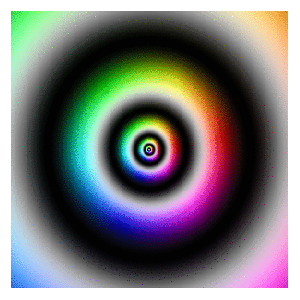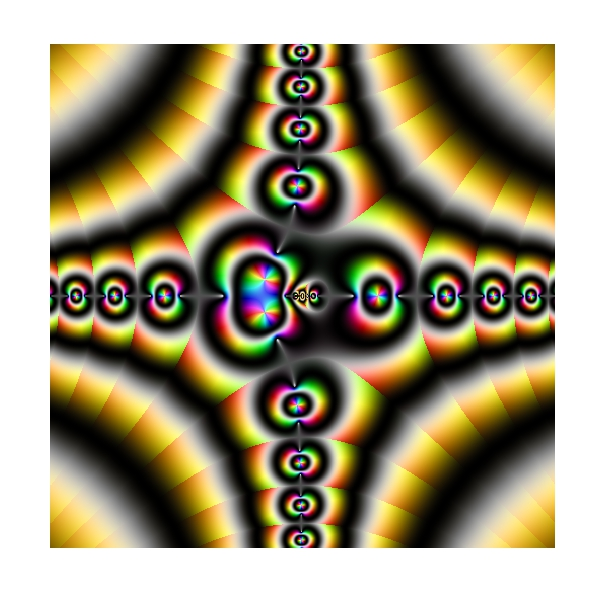# Zooming

You don’t have to be beautiful to turn me on (Kiss, Prince)

I discovered recently how easy is to create GIFs with R using ImageMagick and I feel like a kid with a new toy. To begin this new era of my life as R programmer I have done this:First of all, read this article: it explains very well how to start doing GIFs from scratch. The one I have done is inspired in this previous post where I take a set of complex numbers to transform and color it using HSV technique. In this case I use this next transformation:$f(z)= -Im(z)+(Re(z)+0.5*Im(z))*1i$

Modifying the range of `Real` and `Imaginary` parts of complex numbers I obtain the zooming  effect. The code is very simple. Play with it changing the transformation or the animation options. Send me your creations, I would love to see them:

```library(dplyr)
library(ggplot2)
dir.create("output")
setwd("output")
id=1 # label tO name plots
for (i in seq(from=320, to=20, length.out = 38)){
z=outer(seq(from = -i, to = i, length.out = 300),1i*seq(from = -i, to = i, length.out = 500),'+') %>% c()
z0=z
for (k in 1:100) z <- -Im(z)+(Re(z)+0.5*Im(z))*1i
df=data.frame(x=Re(z0),
y=Im(z0),
h=(Arg(z)<0)*1+Arg(z)/(2*pi), s=(1+sin(2*pi*log(1+Mod(z))))/2, v=(1+cos(2*pi*log(1+Mod(z))))/2) %>% mutate(col=hsv(h,s,v))
ggplot(df, aes(x, y)) +
geom_tile(fill=df\$col)+
scale_x_continuous(expand=c(0,0))+
scale_y_continuous(expand=c(0,0))+
labs(x=NULL, y=NULL)+
theme(legend.position="none",
panel.background = element_rect(fill="white"),
plot.margin=grid::unit(c(1,1,0,0), "mm"),
panel.grid=element_blank(),
axis.ticks=element_blank(),
axis.title=element_blank(),
axis.text=element_blank())
id=id+1
}
system('"C:\\Program Files\\ImageMagick-6.9.3-Q16\\convert.exe" -delay 10 -loop 0 -duplicate 1,-2-1 *.png zooming.gif')
# cleaning up
file.remove(list.files(pattern=".png"))
```

# Visual Complexity

Oh, can it be, the voices calling me, they get lost and out of time (Little Black Submarines, The Black Keys)

Last October I did this experiment about complex domain coloring. Since I like giving my posts a touch of randomness, I have done this experiment. I plot four random functions on the form `p1(x)*p2(x)/p3(x)` where `pi(x)` are polynomials up-to-4th-grade with random coefficients following a chi-square distribution with degrees of freedom between 2 and 5. I measure the function over the complex plane and arrange the four resulting plots into a 2×2 grid. This is an example of the output:Every time you run the code you will obtain a completely different output. I have run it hundreds of times because results are always surprising. Do you want to try? Do not hesitate to send me your creations. What if you change the form of the functions or the distribution of coefficients? You can find my email here.

```setwd("YOUR WORKING DIRECTORY HERE")
require(polynom)
require(ggplot2)
library(gridExtra)
ncol=2
for (i in 1:(10*ncol)) {eval(parse(text=paste("p",formatC(i, width=3, flag="0"),"=as.function(polynomial(rchisq(n=sample(2:5,1), df=sample(2:5,1))))",sep="")))}
z=as.vector(outer(seq(-5, 5, by =.02),1i*seq(-5, 5, by =.02),'+'))
opt=theme(legend.position="none",
panel.background = element_blank(),
panel.margin = unit(0,"null"),
panel.grid = element_blank(),
axis.ticks= element_blank(),
axis.title= element_blank(),
axis.text = element_blank(),
strip.text =element_blank(),
axis.ticks.length = unit(0,"null"),
axis.ticks.margin = unit(0,"null"),
plot.margin = rep(unit(0,"null"),4))
for (i in 1:(ncol^2))
{
pols=sample(1:(10*ncol), 3, replace=FALSE)
p1=paste("p", formatC(pols, width=3, flag="0"), "(x)*", sep="")
p2=paste("p", formatC(pols, width=3, flag="0"), "(x)/", sep="")
p3=paste("p", formatC(pols, width=3, flag="0"), "(x)",  sep="")
eval(parse(text=paste("p = function (x) ", p1, p2, p3, sep="")))
df=data.frame(x=Re(z),
y=Im(z),
h=(Arg(p(z))<0)*1+Arg(p(z))/(2*pi),
s=(1+sin(2*pi*log(1+Mod(p(z)))))/2,
v=(1+cos(2*pi*log(1+Mod(p(z)))))/2)
g=ggplot(data=df[is.finite(apply(df,1,sum)),], aes(x=x, y=y)) + geom_tile(fill=hsv(df\$h,df\$s,df\$v))+ opt
assign(paste("hsv_g", formatC(i, width=3, flag="0"), sep=""), g)
}
jpeg(filename = "Surrealism.jpg", width = 800, height = 800, quality = 100)
grid.arrange(hsv_g001, hsv_g002, hsv_g003, hsv_g004, ncol=ncol)
dev.off()
```

# Complex Domain Coloring

Why don’t you stop doodling and start writing serious posts in your blog? (Cecilia, my beautiful wife)

Choose a function, apply it to a set of complex numbers, paint  the result using the HSV technique and be ready to be impressed because images can be absolutely amazing. You only need `ggplot2` package and your imagination. This is what happens when function is `f(x)=(1+i)log(sin((x3-1)/x))`:To learn more about complex domain coloring, you can go here. If you want to try your own functions, you can find the code below. I will try to write a serious post next time but meanwhile, long live doodles!

```require(ggplot2)
f = function(x) (1+1i)*log(sin((x^3-1)/x))
z=as.vector(outer(seq(-5, 5, by =.01),1i*seq(-5, 5, by =.01),'+'))
z=data.frame(x=Re(z),
y=Im(z),
h=(Arg(f(z))<0)*1+Arg(f(z))/(2*pi),
s=(1+sin(2*pi*log(1+Mod(f(z)))))/2,
v=(1+cos(2*pi*log(1+Mod(f(z)))))/2)
z=z[is.finite(apply(z,1,sum)),]
opt=theme(legend.position="none",
panel.background = element_blank(),
panel.grid = element_blank(),
axis.ticks=element_blank(),
axis.title=element_blank(),
axis.text =element_blank())
ggplot(data=z, aes(x=x, y=y)) + geom_tile(fill=hsv(z\$h,z\$s,z\$v))+ opt
```